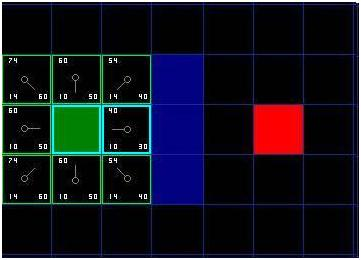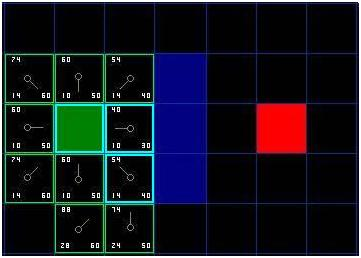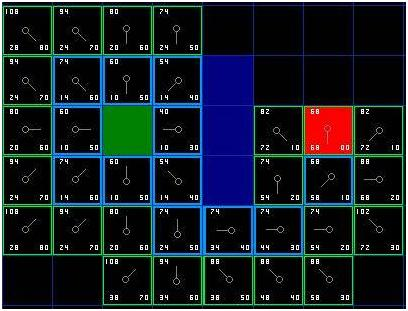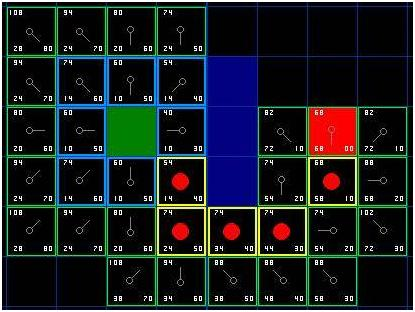## 再译《A *路径搜索入门》之三 原

Continuing the Search

To continue the search, we simply choose the lowest F score square from all those that are on the open list. We then do the following with the selected square:

1.它从开启列表取出，并加入到列表

1.Drop it from the open list and add it to the closed list.

2.检查所有的相。忽略那些列表或不可行走的（，水或其他非法地形），如果它们还不在开启列表中加方开启列表。将定方块作为新方块的“父”。

2.Check all of the adjacent squares. Ignoring those that are on the closed list or unwalkable (terrain with walls, water, or other illegal terrain), add squares to the open list if they are not on the open list already. Make the selected square the "parent" of the new squares.

3.如果相的方开启列表条路那个是否是一个更好的。话说检查，看看方G是否是低，如果我使用当前方到那里。如果没有，什么也不做。

3.If an adjacent square is already on the open list, check to see if this path to that square is a better one. In other words, check to see if the G score for that square is lower if we use the current square to get there. If not, don't do anything.

On the other hand, if the G cost of the new path is lower, change the parent of the adjacent square to the selected square (in the diagram above, change the direction of the pointer to point at the selected square). Finally, recalculate both the F and G scores of that square. If this seems confusing, you will see it illustrated below.

Okay, so let's see how this works. Of our initial 9 squares, we have 8 left on the open list after the starting square was switched to the closed list. Of these, the one with the lowest F cost is the one to the immediate right of the starting square, with an F score of 40. So we select this square as our next square. It is highlight in blue in the following illustration.[Figure 4]

First, we drop it from our open list and add it to our closed list (that's why it's now highlighted in blue). Then we check the adjacent squares. Well, the ones to the immediate right of this square are wall squares, so we ignore those. The one to the immediate left is the starting square. That's on the closed list, so we ignore that, too.

The other four squares are already on the open list, so we need to check if the paths to those squares are any better using this square to get there, using G scores as our point of reference. Let's look at the square right above our selected square. Its current G score is 14. If we instead went through the current square to get there, the G score would be equal to 20 (10, which is the G score to get to the current square, plus 10 more to go vertically to the one just above it). A G score of 20 is higher than 14, so this is not a better path. That should make sense if you look at the diagram. It's more direct to get to that square from the starting square by simply moving one square diagonally to get there, rather than moving horizontally one square, and then vertically one square.

When we repeat this process for all 4 of the adjacent squares already on the open list, we find that none of the paths are improved by going through the current square, so we don't change anything. So now that we looked at all of the adjacent squares, we are done with this square, and ready to move to the next square.

So we go through the list of squares on our open list, which is now down to 7 squares, and we pick the one with the lowest F cost. Interestingly, in this case, there are two squares with a score of 54. So which do we choose? It doesn't really matter. For the purposes of speed, it can be faster to choose the last one you added to the open list. This biases the search in favor of squares that get found later on in the search, when you have gotten closer to the target. But it doesn't really matter. (Differing treatment of ties is why two versions of A* may find different paths of equal length.)

So let's choose the one just below, and to the right of the starting square, as is shown in the following illustration.[Figure 5]

This time, when we check the adjacent squares we find that the one to the immediate right is a wall square, so we ignore that. The same goes for the one just above that. We also ignore the square just below the wall. Why? Because you can't get to that square directly from the current square without cutting across the corner of the nearby wall. You really need to go down first and then move over to that square, moving around the corner in the process. (Note: This rule on cutting corners is optional. Its use depends on how your nodes are placed.)

That leaves five other squares. The other two squares below the current square aren't already on the open list, so we add them and the current square becomes their parent. Of the other three squares, two are already on the closed list (the starting square, and the one just above the current square, both highlighted in blue in the diagram), so we ignore them. And the last square, to the immediate left of the current square, is checked to see if the G score is any lower if you go through the current square to get there. No dice. So we're done and ready to check the next square on our open list.

We repeat this process until we add the target square to the closed list, at which point it looks something like the illustration below.[Figure 6]

Note that the parent square for the square two squares below the starting square has changed from the previous illustration. Before it had a G score of 28 and pointed back to the square above it and to the right. Now it has a score of 20 and points to the square just above it. This happened somewhere along the way on our search, where the G score was checked and it turned out to be lower using a new path – so the parent was switched and the G and F scores were recalculated. While this change doesn't seem too important in this example, there are plenty of possible situations where this constant checking will make all the difference in determining the best path to your target.

So how do we determine the path? Simple, just start at the red target square, and work backwards moving from one square to its parent, following the arrows. This will eventually take you back to the starting square, and that's your path. It should look like the following illustration. Moving from the starting square A to the destination square B is simply a matter of moving from the center of each square (the node) to the center of the next square on the path, until you reach the target.[Figure 7]

（待续）### 如比如比

A*算法之烂片整理

2015/06/14
392
5
Qt相关博客总览

osc_869quh0r
2019/07/24
6
0
《鸡啄米C++编程入门系列》系列技术文章整理收藏

《鸡啄米C++编程入门系列》系列技术文章整理收藏 收藏整理鸡啄米C++编程入门系列文章，供个人和网友学习C++时参考 1鸡啄米：C++编程入门系列之前言 2鸡啄米：C++编程入门系列之一（进制数） ...

2015/05/26
132
0
《鸡啄米C++编程入门系列》系列技术文章整理收藏

2015/06/27
126
0

■实施上的注意事项 Notes on Implementation 现在您了解了基本的方法，当你编写自己的程序时，有一些额外的事情要考虑。下面给出我用C ++和Blitz Basic编写的程序，用其他语言也同样有效。 ...

2015/06/11
76
0

15分钟前
20
0

18分钟前
8
0
vue+elementui实现简易的列筛选功能实现。

21分钟前
42
0

27分钟前
6
0

37分钟前
27
0